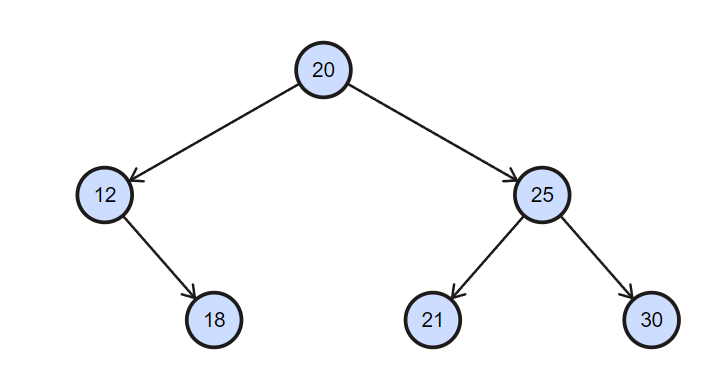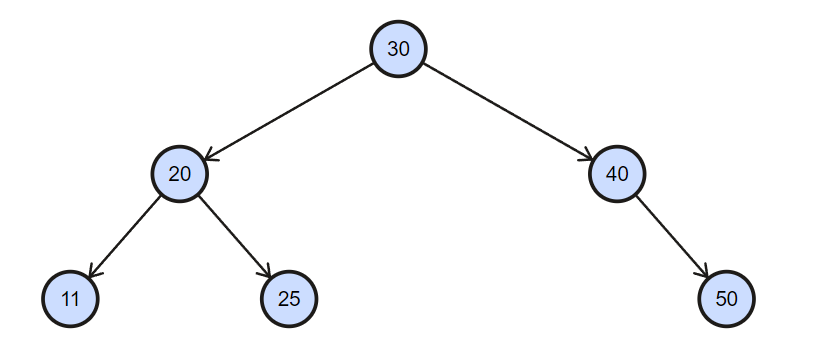# Search in a Binary Search Tree

Problem Statement: Given a root of a Binary Search Tree and the value of a node as X . Find the node that the node’s value equals X and return the subtree with that node and if doesn’t exist return NULL.

Example 1:

```Input: 25Output: 25 21 30```

Example 2:

```Input: 44Output: NULL```

Solution:

Disclaimer: Don’t jump directly to the solution, try it out yourself first.

Solution 1: Using Iterative Method

Intuition:
We know that in BST, if we are currently at a node N, then all the nodes present in the left sub-tree of N will be smaller than N and all the nodes present in the right subtree of N will be greater than N.

If we are at a node N and we want to find X, we can have 3 cases :

1. Value of N == Value of X

In this case, we will simply return the node N.

1. Value of N  < Value of X

In this case, our answer may lie in the right subtree but it’s guaranteed that X is not present in the left subtree because the value of X will always be greater than all nodes present in the left subtree of N.

1. Value of N  > Value of X

In this case, our answer may lie in the left subtree but it’s guaranteed that X is not present in the right subtree because the value of X will always be greater than all nodes present in the right subtree of N.

Approach:

-> We will traverse the Tree using while loop with a condition that root is not equal to NULL and         root’s data is not equal to X

-> If Root’s data is smaller than X,  Root will point to the Root’s right child

-> If Root’s data is greater than X, Root will point to Root’s left child

-> At last when the loop will break, if Root is equal to NULL, means we didn’t get our X so will return NULL, else will return the X node.

Code:

## C++ Code

``````/**
* Definition for a binary tree node.
* struct TreeNode {
*     int val;
*     TreeNode *left;
*     TreeNode *right;
*     TreeNode() : val(0), left(nullptr), right(nullptr) {}
*     TreeNode(int x) : val(x), left(nullptr), right(nullptr) {}
*     TreeNode(int x, TreeNode *left, TreeNode *right) : val(x), left(left), right(right) {}
* };
*/
class Solution {
public:
TreeNode* searchBST(TreeNode* root, int val) {
while(root !=NULL && root->val != val){

root = (root->val > val) ? root->left : root->right ;
}
return root ;

}
};``````

Time Complexity:  O ( log2(N) )    (We won’t traverse in the whole tree )

Space Complexity: O (1)

## Java Code

``````/**
* Definition for a binary tree node.
* public class TreeNode {
*     int val;
*     TreeNode left;
*     TreeNode right;
*     TreeNode() {}
*     TreeNode(int val) { this.val = val; }
*     TreeNode(int val, TreeNode left, TreeNode right) {
*         this.val = val;
*         this.left = left;
*         this.right = right;
*     }
* }
*/
class Solution {
public TreeNode searchBST(TreeNode root, int val) {
while(root !=null && root.val != val){

root = (root.val > val) ? root.left : root.right ;
}
return root ;

}
}``````

Time Complexity:  O ( log2(N) )    (We won’t traverse in the whole tree )

Space Complexity: O (1)

Solution 2: Using Recursive Method

Intuition:

As discussed above, the intuition will be the same. The only difference is we need to think about it recursively. So firstly we need to find what’s the base case?

If we reach Leaf Node or X node, obviously we have to return root from here because there is no scope for further traversal if we hit such a case.

Now, what if we don’t get the above case? It’s simple, we will recursively travel in the tree unless we get the above-mentioned case.

Approach:

-> We will create an extra Node namely as Current to store the current Node we are in.

-> We will check if the root is NULL or the root’s value is equal to X, we will store the root in Current

-> Else if root’s value is smaller than X, we will call recursively to the Right Subtree.

-> Else if root’s value is greater than X, we will call recursively to the Left Subtree.

-> Lastly we will return the Current.

Code:

## C++ Code

``````/**
* Definition for a binary tree node.
* struct TreeNode {
*     int val;
*     TreeNode *left;
*     TreeNode *right;
*     TreeNode() : val(0), left(nullptr), right(nullptr) {}
*     TreeNode(int x) : val(x), left(nullptr), right(nullptr) {}
*     TreeNode(int x, TreeNode *left, TreeNode *right) : val(x), left(left), right(right) {}
* };
*/
class Solution {
public:
TreeNode* searchBST(TreeNode* root, int x) {
TreeNode * current;
if (root == NULL || root -> val == x)
current = root;
else
if (root -> val < x)
current = searchBST(root -> right, x);
else
if (root -> val > x)
current = searchBST(root -> left, x);

return current;

}
};``````

Time Complexity:  O( log2(N) )    (We won’t traverse in the whole tree)

Space Complexity: O(N)      (worst space complexity because we are using Recursion)

## Java Code

``````/**
* Definition for a binary tree node.
* public class TreeNode {
*     int val;
*     TreeNode left;
*     TreeNode right;
*     TreeNode() {}
*     TreeNode(int val) { this.val = val; }
*     TreeNode(int val, TreeNode left, TreeNode right) {
*         this.val = val;
*         this.left = left;
*         this.right = right;
*     }
* }
*/
class Solution {
public TreeNode searchBST(TreeNode root, int x) {
TreeNode current=null;
if (root == null || root.val == x)
current = root;
else
if (root.val < x)
current = searchBST(root.right, x);
else
if (root.val > x)
current = searchBST(root.left, x);

return current;

}
}``````

Time Complexity:  O ( log2(N) )    (We won’t traverse in the whole tree )

Space Complexity: O (N)      (worst space complexity because we are using Recursion )